# L-function

(diff) ← Older revision | Latest revision (diff) | Newer revision → (diff)

A generalization of the zeta-function at the cost of introducing characters (cf. Character of a group). The-functions form a complicated class of special functions of a complex variable, defined by a Dirichlet series or an Euler product with characters. They are the basic instrument for studying by analytic methods the arithmetic of corresponding mathematical objects: the field of rational numbers, algebraic fields, algebraic varieties over finite fields, etc. The simplest representatives of-functions are the Dirichlet-functions (cf. Dirichlet-function). The remaining-functions are more or less close analogues and generalizations of these-functions.

Nowadays-functions comprise a very large class of functions which are attached to representations of the Galois group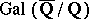. For example, choose a representation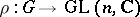of the Galois groupof an algebraic number field(cf. Representation of a group). For each prime, let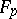be a Frobenius element in. Then the function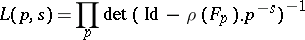is the Artin-series corresponding to. In a similar way, the action ofon the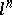-torsion points of an elliptic curve, defined over, gives rise to the Hasse–Weil-function of. There exists a large body of fascinating conjectures about these-functions, which, on the one hand, relate them to automorphic forms (Langlands' conjectures) and, on the other hand, relate values at integral points to algebraic-geometric invariants (Beilinson's conjectures).